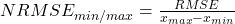# SNOWFALL BUDDY

## Snowfall and Rainfall Estimation Tool

### Online Tool

→ 2. Background
→ 3. User Guide
→ 3.1. Quick start
→ 3.2. Home Module
→ 3.3. Input Data Module
→ 3.4. Analysis Module
→ 3.5. Output Timeseries
→ 3.6. Calibration Procedure
→ 3.7. Data inspection, visualisation and export
→ 4. Limitations
→ 6. Contact

• ### 2. Background

##### Depending on the ambient air temperature, precipitation can fall as rain, snow or as a mix of rain and snow. Hence, for cases when the snowfall data is not directly available, the snowfall portion of the total precipitation is estimated using the following formula: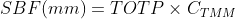##### Consequently, the rainfall amount is estimated as the difference between TOTP and SNF (mm) using the following formula: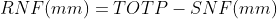##### The amount of snowfall expressed as centimeters of snow layer, that corresponds to the amount of snowfall expressed as millimeters of water is also depending on the ambient air temperature and can be estimated using the following formula: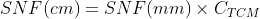• ### 3. User Guide

#### 3.3. Input Data Module

##### The number of columns, data format and the units of the various timeseries required for the input file are shown in the table below.
 Columns Units Values SNOW BUDDY Input data file: format, columns, and units for input and user calibration data (UCD) DATE TEMP TOTP UCD (max. 3 columns) yyyy-mm-dd °C mm user choice eg. 2021-12-24 -70 to 50 0 to 1800 user choice

#### 3.5. Output Timeseries

##### The output from SNOWFALL BUDDY is shown in the table below.
 NAME DESCRIPTION Index Indicates the position of a specific record (line) in the timeseries. SNOW_MM (mm) Amount of snowfall expressed as millimeters of water. SONW_MM is obtained using TOTP (total precipitation) and CTMM (air temperature coefficient for conversion of total precipitation to snowfall). SNOW_CM (cm) Amount of snowfall expressed as centimeters of snow. SONW_CM is obtained using SNOW_MM (snowfall expressed as millimeters of water) and CTCM (air temperature coefficient for conversion of snowfall from mm of water to cm of snow). RAIN_MM (mm) Amount of rainfall. RAIN_MM is calculated as the difference between TOTP and SNOW_MM.

#### 3.6. Calibration Procedure

##### To aid with data inspection and assessment of the fitness of the output data, SNOWFALL BUDDY includes several univariate and bivariate statistics. Univariate statistics, including, average, minimum, maximum and standard deviation are calculated separately for the input (i.e., user provided calibration data) and tool output time series. The graphs and the univariate statistics can be used for example for comparing the general trends, the range of values and the amplitude of variations in both data sets. The bivariate statistics include the coefficient of determination (R2), root mean square error (RMSE) and the normalized root mean square error (NRMSE). NRMSE is calculated by using the average, the interquartile range or the differences between maximum and minimum (see definitions below). The bivariate statistics are used for evaluating the fitness of the output, by providing a measure of the differences between the values calculated by the tool and the user provided calibration data (i.e., UCD). The equations used for calculating each bivariate statistic are shown below.##### Eq 4. - Coefficient of determination
###### R2 - coefficient of determination xi - value for observed data on day i yi - value for modelled data on day i xmean - mean of observed data##### Eq 5. - Root mean square error
###### N - number non-missing data points xi - value for observed data on day i yi - value for modelled data on day i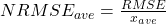##### Eq 6. - Normalized root mean square error average
###### NRMSEave - normalized root mean square error calculated using the average value of measured data RMSE - root mean square error xa - average value of observed data##### Eq 7. - Normalized root mean square error IQR
###### NRMSEIQR - normalized root mean square error calculated using minimum and maximum values of measured data RMSE - root mean square error IQR - interquartile range of the observed data; IQR = Q3-Q1,with Q3 = CDF-1(0.25), Q1 = CDF-1(0.75),where CDF is the quantile function